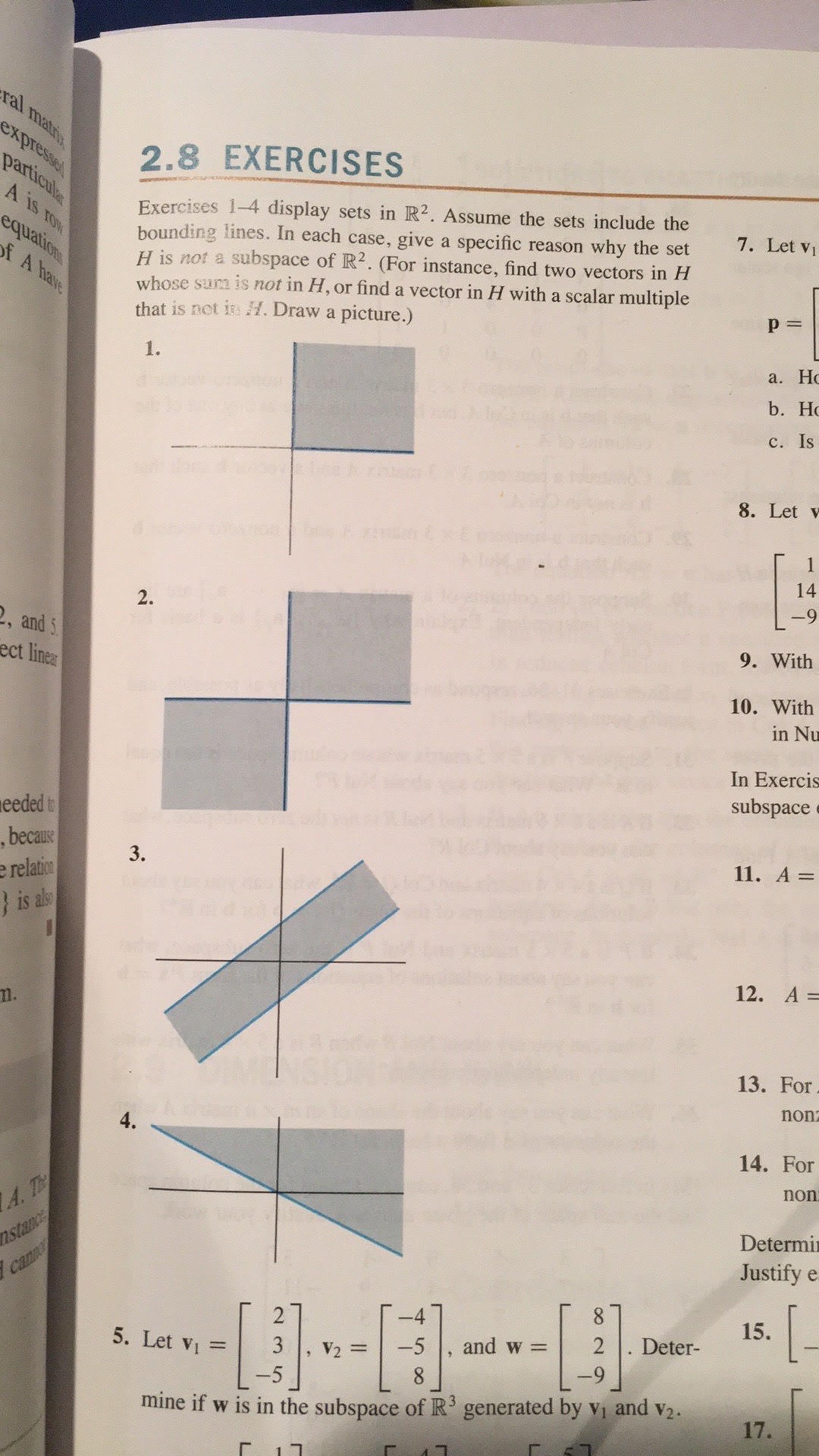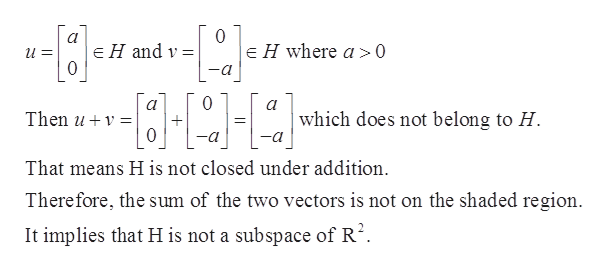# ral matixexpresseparticuleEXERCISES2.8A is TONExercises 1-4 display sets in R2. Assume the sets include thebounding lines. In each case, give a specific reason why the setH is not a subspace of R2. (For instance, find two vectors in Hwhose sura is not in H, or find a vector in H with a scalar multiplethat is not i H. Draw a picture.)7. Let viequationf A haveр3D1.а. Ноb. Hос. Is8. Let v1492.2, and 5ect linear9. With10. Within NuIn Exercissubspaceeeded t, becausee relation} is als11. А 3D3.12. А -13. Fornon4.14. FornonA.TnstancecanDetermiJustify e15.Deter-2and w =5. Let vi-53V28-5mine if w is in the subspace of R generated by vi and v2.17.

Question
6 views

I don't understand how to do problems 2,3,4.help_outlineImage Transcriptioncloseral matix expresse particule EXERCISES 2.8 A is TON Exercises 1-4 display sets in R2. Assume the sets include the bounding lines. In each case, give a specific reason why the set H is not a subspace of R2. (For instance, find two vectors in H whose sura is not in H, or find a vector in H with a scalar multiple that is not i H. Draw a picture.) 7. Let vi equation f A have р3D 1. а. Но b. Hо с. Is 8. Let v 14 9 2. 2, and 5 ect linear 9. With 10. With in Nu In Exercis subspace eeded t , because e relation } is als 11. А 3D 3. 12. А - 13. For non 4. 14. For non A.T nstance can Determi Justify e 15. Deter- 2 and w = 5. Let vi -5 3 V2 8 -5 mine if w is in the subspace of R generated by vi and v2. 17. fullscreen
check_circle

Step 1

We’ll answer the first question (That is 2nd question you asked). Please submit remaining questions again one by one.

2.Consider the given diagram which represents the set H in R2

Step 2

Assume the sets included the bounding lines.

Now, H will be a subspace of R2 if,

i).The zero vector is in H.

ii).For each u and v in H, the sum of u and v should be in H.

iii).For each u in H and scalar c, cu should be in H.

Step 3

By the given diagram it is obvious that zero vector is in H.

Now consi...help_outlineImage Transcriptionclose0 E H where a>0 E H and v = 0 a 0 а which does not belong to H Then u 0 -a That means H is not closed under addition Therefore, the sum of the two vectors is not on the shaded region It implies that H is not a subspace of R2. fullscreen

### Want to see the full answer?

See Solution

#### Want to see this answer and more?

Solutions are written by subject experts who are available 24/7. Questions are typically answered within 1 hour.*

See Solution
*Response times may vary by subject and question.
Tagged in

### Math